# Amazon.com — Employee Access ChallengePhoto taken from Kaggle

# Table of Contents

1. Problem statement

# 1.Problem statement:

Predict an employee’s access needs, given his/her job role.

# 2.Problem Description:

When an employee at any company starts work, they first need to obtain the computer access necessary to fulfill their role. This access may allow an employee to read/manipulate resources through various applications or web portals. It is assumed that employees fulfilling the functions of a given role will access the same or similar resources. It is often the case that employees figure out the access they need as they encounter roadblocks during their daily work (e.g. not able to log into a reporting portal). A knowledgeable supervisor then takes time to manually grant the needed access in order to overcome access obstacles. As employees move throughout a company, this access discovery/recovery cycle wastes a nontrivial amount of time and money.

There is a considerable amount of data regarding an employee’s role within an organization and the resources to which they have access. Given the data related to current employees and their provisioned access, models can be built that automatically determine access privileges as employees enter and leave roles within a company. These auto-access models seek to minimize the human involvement required to grant or revoke employee access.
The above problem statement has been taken from Kaggle .

# 3.Data Overview

Taken data from Amazon.com — Employee Access Challenge on kaggle https://www.kaggle.com/c/amazon-employee-access-challenge The data consists of real historical data collected from 2010 & 2011. Employees are manually allowed or denied access to resources over time. You must create an algorithm capable of learning from this historical data to predict approval/denial for an unseen set of employees. — Data columns (total 10 columns):

# 4. Mapping the problem into supervised learning problem:

Generated new features like Pair wise column featurization. I have considered pairs of 2 columns as well as pairs of 3 columns, and analyzed if these feature pairs help to improve the model prediction. Also generated new features using Frequency encoding and Target encoding. Later I have used all these features and train various ML model like Logistic Regression, Random Forest, XGBoost.

# 5. Business objectives and constraints:

• The objective of this competition is to build a model, learned using historical data, that will determine an employee’s access needs, such that manual access transactions (grants and revokes) are minimized as the employee’s attributes change over time. The model will take an employee’s role information and a resource code and will return whether or not access should be granted.

# 6. Performance metric for supervised learning:

AUC (As mentioned in the competition evaluation criteria)

AUC Explanation -

AUC is an important metric for Binary classification. Here’s is how ROC-AUC works:

→ First , the dataset is sorted by the value of y-hats(predicted probabilities).

→ If there are “N” number of samples, then there are “N” thresholds.

→ Then False Positive Rate (FPR) and True Positive Rate(TPR) is calculated for each of the Thresholds. Both the TPR and FPR for all the thresholds lie between 0 to 1

→ All these pairs of TPR and FPR are plotted. The plot looks similar to this: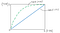Self made drawing

A Random model would predict AUC = 0.5(as shown by the Blue Line). For a sensible model, The Roc-AUC value will be greater than 0.5 (as shown by the dotted Green curve). If the AUC is lesser than 0.5, then the model is said to be worse than the Random model.

Quest: What are TPR and FPR ?
Ans: TPR and FPR are the ratios that we get from a confusion matrix.
TPR means — out of all the points that are actually Positive , how many of them are correctly predicted as Positive.
FPR means- out of all the points that are actually Negative, how many of them are incorrectly predicted as Positive

# 7. EDA:

## 7.1First let’s load the train data and check the number of data points and features

Result of the above cell

## 7.2 Get the total categories present in each column of the dataframe

If we closely observe, “ROLE_TITLE” and “ROLE_CODE” have exactly the same number of categories. Hence there is a possibility that every unique ROLE_TITLE is mapped to an unique ROLE_CODE. Let’s investigate this a little further

Let’s drop the duplicate rows across ‘ROLE_TITLE’and ‘ROLE_CODE’ except the first occurrence of a particular category and get the number of Unique ‘ROLE_TITLE’ and ‘ROLE_CODE’.

IF the Unique values is still 343 for both ‘ROLE_TITLE’ and ‘ROLE_CODE’, then it means that there is a one to one mapping

Output of the above cell:

Since there is a one-to-one mapping , we can drop either ‘ROLE_TITLE’ or ‘ROLE_CODE’.

Note: In the drop_duplicates code, inplace = False by default, this means that the original dataframe still remains unchanged

## 7.3 Check for Nan values and Count of each class in dataframe:

There are no NaN values in the dataframe.
Now check for the Count of each class in the dataframe. This will tell us if the dataset is balanced or imbalanced.

Output:

This is a highly imbalanced data. Any dummy dumb model which always predicts class = 1 on the train data, will give an accuracy of 93 %. This means that only if the model that we would build, gives an accuracy more than 93%, then only we can say that the ML model has learned something

## 7.4 Pair Plots-

From the pair-plots as well, it’s quite evident that the data is not separable with the current features.

Output of the above cell (you can download the image and zoom-in using your image viewer for better visibility):

From the above plots ,I did not find any pdf-plot or scatter plot which separates the 2 classes.

Note that the type of data here is Categorical, and not Numerical. Later, we will have to do One-Hot-Encoding using CountVectorizer. But the CountVectorizer expects the data to be text data. Therefore , we need to explicitly convert the columns into Type: Str

We do the same operation with test data as well

## 7.5 Let’s see TSNE plot on the train data and check if TSNE can separate the data based on the original features of the dataset

I have written a function for TSNE which I can call later to show the plot.

Lets call the TSNE function.

We can run TSNE on different perplexity values and check the plot if there is any separation and consistency. E.g- TSNE shows class separation at perplexity = 30 as well as 50, then we can say that TSNE’s output is consistent and trusted.

As we can see, in both the plots, there are multiple cluster being formed and the class labels are not getting separated. Therefore, TSNE on the original data could not separate the classes.

# 8. Feature Engineering

## 8.1 Feature Engineering(Pair-Wise featurization)

We will create new features based on pair-wise combination of the original column. We will create pairs of degree 2 and degree 3 in both Train and Test data.
Therefore the total number of columns formed should be 8C2 + 8C3 + 8 = 92

The formula for calculating combination is as follows: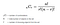Image taken from mathplanet.com

For more information , visit this Link

So I have created 2 functions to create pairs of degree 2 and degree 3.

Also, we can go for more higher degrees if we wish. But this means more features and time complexity of the ML models will increase. So , we will only use degrees 2 and 3.

Lets plot TSNE for this new feature set

Now it looks like the data is taking some shapes and the label 0 (blue points) are at the periphery of the circular shape formed. We get the same shape in all the above 2 TSNE plots. So, the data is a little more interesting now.

Like we created Pair-Wise featurization for Train data, we will do the same for the Test data that we got from Kaggle

## 8.2 Feature Engineering(Mean or target encoding)-

Mean or target encoding as a form of encoding in categorical variables. Mean encoding implies replacing the categorical values by the average target value for that specific category. An advantage of this type of feature engineering is that It does not expand the feature space. But there is 1 disadvantage of Target encoding: If 2 categories show the same mean of target, they will be replaced by the same number and this may lead to potential loss of information. This disadvantage also exists with frequency encoding as well. E.g.- Mean Encoding of Red will be (1+0)/2 = 0.5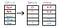Self made Image

## 8.3 Feature Engineering(Count or Frequency Encoding)-

Count or Frequency Encoding implies replacing the categories of a categorical variable with the count or percentage of observation that the specific category has in the dataset. It shows how frequent / predominant that category is. Again, the advantages and disadvantages are same as that of Target Encoding. E.g.- Frequency of Red will be 2/5 = 0.4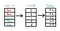Self made Image

Helpful for Model productionization — While doing Frequency encoding and Mean encoding on train data(consisting of 92 column-after doing Pairwise featurization), it’s essential to store the encodings for each individual category for all the 92 Columns. We need to create a dictionary with key as the column name and value as the frequency encodings of the various categories of that column. This means that there will be 92 key-value pairs in the dictionary. Finally , save this dictionary as a pickle file. I will discuss more about this in Section 12.

## 8.4 Feature Engineering(CountVectorization /TF-IDF + SVD)-

We will get the Bag of Words (BOW) representation of each and every column (92 columns) using CountVectorizer. For this reason, the columns were converted to string type earlier. After doing CountVectorization, the size of the data will expand abnormally. Remember the amount of categories that were present in the original data for each feature ? (Ans: Refer point 6.2). And additionally we did pair-wise featurization. Therefore the expansion in dimension will be amazingly large. Therefore we will do something special here. After doing CountVectorization on each feature, we will shrink the expansion back to just one feature. To do this we will use SVD with n_components = 1.
Therefore, CountVectorization+ SVD will be applied on each and every 92 columns. The result is that the final dimensionality of the data is still 92 but the data gets transformed.

Also, instead of doing CountVectorization+ SVD , we can also do TF-iDF + SVD. Infact we will go with TF-iDF + SVD because it gave better results in kaggle.

Helpful for Model productionization — Save each of the 92 TF-IDF Vectorizer after fitting it on each of the 92 column of the train data. Remember that we did TF-IDF Vectorization on each of the 92 columns(got 92 columns after doing Pairwise featurization) and then initialized TruncatedSVD object(with “n_component = 1”) and fitted on each of these TfidfVectorizer objects . This also means that we need to save each of the 92 TruncatedSVD objects after fitting it on each of the 92 TF-IDF Vectorizer.

Save all the TF-IDF Vectorizers in a list and convert it into a pickle file. Do the same for all TruncatedSVD objects. We will look more into this in Section-12.

## 8.5 Concatenate the result of Points 8.2, 8.3, 8.4 -

Now , it’s time to concatenate the feature sets that we created in Section 8.2, 8.3, 8.4 . Remember, that in each of these sections, the result has a dimensionality of 92 columns. Therefore, after concatenation, the result will have a dimensionality of 276 columns. This dimensionality is quite large to apply TSNE on. Therefore we will not be able to do TSNE here anymore.

# 9. Explanation for Bag Of Words (BOW), TF-IDF, Singular Value Decomposition(SVD)

## 9.1 Bag Of Words (BOW) -

It’s a technique to convert text to vector. Here are the steps to get BOW representation.

Step 1- construction of dictionary- Set of all words in your corpus. Here each unique word is like a unique category. Here, a dictionary can be thought of as the bag that contains each unique word.
Step 2- Now vectorize each sentence. For a given sentence, this will place the number of occurrences of a given word in the cell which is assigned for this word. But this leads to the creation of sparse vector for each review or text

The following diagram shall make the concept more clear. You can think of this as, there is a text feature / column and we want to get the vector representation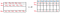Self made Image

Here W₁ , W₂, W₃ , W₄, W₅, W₆, W₇ are the words present in the dictionary. Sent₁, Sent₂….are the Sentences present in the feature / Column. Therefore, we can see that each sentence has been transformed to it’s vector representation..

Note: There are different types of BoW:

1. Unigram — Each unique word is considered a dimension. Disadvantage — Doesn’t make use of the sequence information. Often, sentences that have subtle differences and are exactly opposite (e.g- Sentence 1 :This house is very large , Sentence 2: This house is not large.) are given almost the same vector representation inspite of being just opposite in semantic meaning.

In this Case Study, we have to consider only unigram BOW because our data is categorical text/word and is not a sentence.

## 9.2 TF-iDF-

TF-iDF stands for Term Frequency- Inverse Document Frequency

Term frequency is what is the probability of finding a word in a document

Example — If TF(wᵢ , reviewⱼ) = ⅖ ; where 2 is the no. of times wᵢ has occurred in reviewⱼ and 5 is the total words in the reviewⱼ

IDF- Inverse Document Frequency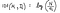Self made Image

Here D𝒸 is the total Document Corpus ; N is the number of documents in the corpus and nᵢ is the no. of times Wᵢ has occurred in the Corpus

If word is more frequent , then the IDF will be low and if the word is rare , then the IDF will be High

Ques: How to calculate the TF-IDF for a word in a given review ?

Ans: TF-IDF of (Wⱼ , Rᵢ ) = TF(Wⱼ , Rᵢ ) * IFD(Wⱼ , D𝒸 )
So if IDF is high, i.e.- Word is rare in the corpus; and TF is high, i.e.- Word probability is high in that review; then we can say that the TF-IDF will be high. This means that particular word will have more weightage in the given review / sentence.

Advantage of using TF-IDF — Just like Bag of Words, TfidfVectorizer also has the parameter called “ngram_range” wherein we can specify the the range of n-grams we want to have in the dictionary

Disadvantage of using TF-IDF — TF-IDF will not consider the semantic meaning of words. I.e.- Tasty and Delicious. Both the words will be considered as completely different words with no semantic similarity.

## 9.3 SVD — Singular Value Decomposition

SVD is a Matrix Factorization technique.
Just like 10 = 5 * 2 , which means that 10 has factors of 5 and 2. We will use the same theory in Matrices as well. I.e.- if we have a Matrix V , then we can write V = A*B*C

Even PCA, which is a classic Dimensionality Reduction technique is also based on Matrix Factorization. In PCA, we have to first calculate the Covariance matrix and then we do factorization / decomposition on top of this covariance matrix.

Now , dimensionality reduction means reducing the dimensions of a given matrix. Therefore, if we want to reduce the dimension (d) to just 5, then we will have to take the EigenVectors corresponding to the top 5 EigenValues.

But in SVD, we do not have to calculate the covariance matrix. We can directly do factorization / decomposition on top of the original matrix.

Here X is the Original matrix which gets decomposed into 3 different matrices. In other words, these 3 matrices are the factors of X .

Here 𝜮 is the diagonal matrix where the principal diagonal consists of the singular values .

U is called the Left Singular matrix .

V is the Right Singular matrix.

And then , the story goes the same as that of PCA. In order to reduce the dimensionality(d) to just 1 , we take the Singular matrix corresponding to the top Singular value

Now coming to our case study. I have applied TF-IDF vectorization for a given Column and on top of this I have applied SVD with n_component = 1

TF-IDF vectorization of a column will result in a vector of shape n x d where “n” is the number of rows and “d” is the total number of categories in that column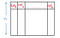Self made Image

Now using SVD we get the best component out of all the d components above.

We apply TF-IDF + SVD on all the Columns in the dataframe.

This means that if the dataframe has 92 columns, then after doing TF-IDF + SVD on all the columns in the dataframe , the result will also have 92 columns. This means that we are able to get better data representation without increasing the dimension of the data.

Therefore after performing all the Feature Engineering techniques, we finally have train and test dataset which have 92 columns each. Later we will use this train dataset and train various Machine Learning models on top of it.

# 10. Machine Learning Models:

## 10.1 Logistic Regression -

Logistic Regression is a classical ML algorithm that works on the assumption that the data classes are almost / perfectly linearly separable.
Task- The task of Logistic Regression is to find the hyperplane 𝝥 that best separates the Positive points from the Negetive points.
In that case , the plane that separates the positive points from the negative points can be represented by the equation — 𝝥: Wᵀ X + b : where W is a vector that is normal to the plane and b is the intercept.

Remember that the Weight Vector W here is a D dimensional vector. D is the dimension of every datapoint Xᵢ

Weight Vector( W ) = <W₁ , W₂, W₃ , W₄ , …..W𝒹>

So corresponding to every feature, I have a weight associated with it. This tells us how important a feature is. This increases the interpretability of the model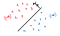Self made drawing

Let’s display the positive labeled points (actual class) as +1 (i.e. Yᵢ = 1) and negative labeled points (actual class) as -1(i.e. Yᵢ = -1). Now let’s say that we want to calculate the distance of a red point Xᵢ from the Plane 𝝥. Let’s name this distance as dᵢ .

Now dᵢ = (W Xᵢ ) / || W ||

Since W is a unit vector, so || W || is equal to 1. Therefore dᵢ = W Xᵢ

Now dᵢ > 0 if both W and Xᵢ lie in the same side of the plane. Else it will be < 0

For both Positive and Negative labelled points (actual class), if Yᵢ * (W Xᵢ ) > 0 , then we can say that the model has correctly predicted the class of that point. Otherwise it’s a misclassification.

The optimization problem for Logistic Regression would be:

Which means , find that W★ that maximizes the Sum of Yᵢ * (W Xᵢ ) . In order for this to be maximum, we want as many positive values of Yᵢ * (W Xᵢ ) as possible.

Ques: How does Logistic Regression deal with Outliers ?

Ans: Remember that every single outlier can greatly affect the Model. For the outlier points , the signed distance is very large. Therefore they impact the model’s decision to a large extent. In such a case the model might select a bad hyperplane inorder to maximize the above equation. This means that the max sum of signed distance is not prone to the outliers (meaning that the Outliers can impact the model a lot). The solution to this is Squashing.
Squashing brings in more conditions to the concept of Sum of Signed distance.
Squashing says that if the Signed distance of a Point is small→ then use it as it is in the equation of Sum of Signed distance.
But if the Signed distance of a Point is large → Squash the value and make it smaller.
Therefore the Sum of Signed distance equation has to be passed through a function that does this squashing. One such function is called Sigmoid function (represented as 𝞼).

The above equation for 𝝥 is less impacted by outliers than the Sum of Signed distance equation.

This equation can be re- written as

Where the log() term is the loss term

Remember: The least value that the above equation can yield is 0.

Overfitting and underfitting in Logistic Regression : In order to minimize the above equation, the Logistic Regression algorithm can get lured to make the Wᵢ’s → ∞ in the vector W .The model can also be tempted to correctly predict all the training data points and thus making ( yᵢ W Xᵢ ) maximum. This condition will lead to Overfitting where the model performs very well on train data but there is no guarantee that the model will work well on inference data. Therefore to avoid this problems , we can modify the equation and add Regularization

Where 𝝺WᵀW is the regularization term.

This can again be re-written as 𝝺 ||W||²₂

Lambda(𝝺) is the hyperparameter of Logistic Regression. The above equation is L2 norm regularization

Now, the above modified equation will prevent Wᵢ’s → ∞

Ques: How does changing Lambda(𝝺) effect the LR model ?

Ans: If Lambda(𝝺) is large, then the influence of the Loss term is not significant anymore which inturn means that the model is no longer making use of the training data (xᵢ’s and yᵢ’s). This condition will lead to Underfitting where the model will perform poorly even on the Train data.
If Lambda(𝝺) is small, then the influence of Regularization term is not significant anymore, which means than now Wᵢ’s → ∞ inorder to minimize the above equation and this will lead to Overfitting.

Ques: What is L1 Regularization in Logistic Regression and how does it affect it ?

Ans: L1 regularization is an alternative to L2 Regularization. The L1 regularization term will be ( 𝝺 ||W||₁ )where 𝝺 is again the hyperparameter. L1 regularization is used to create sparsity. In the case of Logistic Regression, L1 regularization will simply check for the smaller Wi’s in the Weight vector <W₁ , W₂, W₃ , W₄ , …..W𝒹> and make them Zero. L1 regularizer can be used when the dimensionality is very large and we want to simply remove the unnecessary feature weights. This can also improve the latency as the model would have lesser computation to perform.

Train and run time complexity:

Train time complexity is O( n * d); n= number of train points, d= number of features.

Run time Space complexity = O(d); d= number of features. Reason- After training, the model will only need to store the best W vector which is equal to <W₁ , W₂, W₃ , W₄ , …..W𝒹> . Therefore Run time Space complexity does depend on “d”.

Run time Time Complexity = O(d); d= number of features. Reason- During run time, to determine whether the test point(of dimension d) is positive or negative , the model will simply do a dot product of the stored W vector with the Test point. Run time Time Complexity will again depend on the dimensionality “d” ..

Ques: So under what circumstances can I use the Logistic Regression algorithm ?

Ans: Remember the assumption for Logistic Regression? — data classes are almost / perfectly linearly separable. But in real world problems , the data might be complex and just one linear decision surface might not be sufficient enough for separating the data. Therefore regularization can be used for Binary Classification but might not be the best model. But if I want model interpretability, then LR is a very good option(Reason- the weights associated with each feature does tell me about feature importance).

Note:

→ Before going for Logistic Regression implementation, make sure that all the features are Column standardized. Reason- the features might be on different scales and since the algorithm calculates distances between points and the hyperplane, it’s important to bring all features to the same scale.

→ If there is collinearity / Multicollinearity in the dataset, then the feature weights cannot be used as feature importance.

E.g — if feature₁ = 𝛂₁ + 𝛂₂(feature₂) + 𝛂₃(feature₃) ; then we can say that feature1 ,feature2 ,feature3 are multicollinear. Therefore, it’s always safe to first check if there is multicollinearity in the dataset- using techniques like Pertubation

Now Let’s take the train dataset that we formed using feature engineering (Refer Section-9), and apply Logistic Regression for training

In the above code, I am doing Hyperparameter tuning using GridSearchCV (with cross-validation splitting strategy as stratified k-fold cross-validation). StratifiedKFold() ensures that classes are equally distributed among each folds- In our case number of folds = 5.

Note that I have used SKLearn’s SGDClassifier with loss=‘log’. This will behave same as Logistic Regression. Also, I have used class_weight=‘balanced’ which will help slightly in the case of imbalanced dataset. This is a way to give more weightage to the minority class samples.

The result of the above code is as follows:

Let’s simply print the best estimator

Next , let’s check the mean, min and max train score and cv score (here it is denoted as test score)

Output of the above code:

We have got a pretty good mean cross validation score of 0.883

## 10.2 Decision Trees:

Decision trees are simply Nested If-Else conditions.
There are 3 essential concepts that will help us understand Decision trees.

A- Entropy , B- Information Gain , C- Gini Impurity. Let’s understand these 3 concepts one by one.

A- Entropy(H): the Entropy of this random variable Y is mathematically defined as :

Where K = Number of classes

Suppose we are given a random variable Y which take only 2 values — +1 and -1 , then the plot for the entropy will be:

This means that if both the classes (+1 and -1) are equally-probable (Probability — P = 0.5 for both the classes), then the Random variable Y will have the highest Entropy of 1. 1 is the maximum entropy possible. The entropy is minimal when one class is most probable and for rest of the classes it’s close to 0.

Decision tree splits in such a way that the Entropy at the subsequent level (child nodes) decreases and Information Gain Increases. So a Decision Tree checks for every feature and then selects splitting at that feature which gives maximum Information Gain.

Suppose, we have a dataset D and the Decision Tree decides to split by feature f₁ , supposing feature f₁ has 2 categories, therefor the split will look like:

Suppose that the Root node with dataset D has an entropy of H(D), entropy for the dataset D₁ is H(D₁) and entropy for the dataset D₂ is H(D₂) , then the Information Gain would be:

(IG)for 𝒻₁ = H(D) — [ Weighted sum of Entropy after breaking the dataset into D₁ and D₂ ]

Weighted sum of Entropy will be the (Ratio of Points in D₁,* H(D₁) ) + (Ratio of Points in D₂* H(D₂) )

In the same way, the decision tree will check the splitting on feature f2 , f3 , ….. Fn and calculate (IG)for 𝒻₂, (IG)for 𝒻₃ ……….(IG)for 𝒻ₙ ,. From these IG’s the algorithm will select the one that is the highest and the corresponding feature is the ideal feature on which splitting should be done. This same idea is used for splitting across nodes at the child levels.

Like Entropy, there’s a similar idea called Gini Impurity (I𝓰).

Mathematically I𝓰 is represented as :

The plot for I𝓰 (Gini Impurity ) and H (Entropy) can be together shown as:

The red plot is for I𝓰 (Gini Impurity ) and the Blue plot is for H (Entropy) . As can be seen, the max value for I𝓰 is 0.5 and occurs when the classes are equally-probable.

Ques: So if the Gini Impurity behaves similar to Entropy then why can’t we use gini impurity instead of entropy, to decide on which feature to do splitting ?

Ans: True, Scikit-Learn actually uses the Gini Impurity concept. As we can see, the equation of Gini Impurity is quite straightforward and unlike Entropy, it does not use logarithm in it’s equation. Hence the time complexity for calculating Gini Impurity will be much lesser than that of Entropy. That’s the reason Scikit Learn uses Gini Impurity instead of Entropy for deciding the splitting.

Let’s move forward and see how the decision tree does classification

In a decision tree, each node has the power to make decision. Note that I don’t want the bottom-most node to make decisions based on a small number of points / 1 point. This is because there is a high chance that these points are noisy points. Points in a node become lesser as depth increases. As depth of the tree increases, chances of overfitting to noise increases. Also , if the depth of the tree is small , then it may lead to Underfitting. Also, if depth increases, then it becomes harder to understand what is happening in a decision tree and therefore interpretability decreases as Depth increases.

We stop grow the tree in 3 cases:

1. When the algorithm reaches pure nodes. In that case , the algorithm will no longer split that node.

Overfitting and Underfitting: The Decision Tree will tend to Overfit if the Depth is Large. In this scenario, there is a high chance that the Tree will overfit to the noisy data. On the other hand, if the depth is low (e.g- If depth = 1) then the model is not trained to its fullest potential and this scenario will lead to underfit, where the model will perform poorly even on the train data

Train and Run time complexity of Decision Tree-

Train time Complexity is O( N(log₂N )d ) ; where “N” is the number of data-points in the training dataset and “d” is the number of dimensions/features

If depth is kept reasonable , then the Run time Space complexity is reasonable.

Time complexity at Run time is O(depth of the Decision Tree)

After training is complete, just store the DT, which is a nested If-Else statement.

Therefore, the Space Complexity at Runtime is : Number of Internal nodes and the number of Leaf nodes

Ques: So under what circumstances can I use Decision tree algorithm?

Ans: We can use DT when the dataset is large and dimensionality is reasonable / small. DT can also be used in systems which demand low latency. Reason- The Runtime time complexity is just O(Depth of Decision Tree). Also we can use Decision Tree on case studies which demand good model interpretability. Reason- Decision Tree are just nested If-Else statements which are easily interpretable if the depth is small.

For this Cases Study, instead of using Decision Tree, I have used Random Forest which we will visit in the next section (Section-10.3)

## 10.3 Random Forest

Before learning Random Forest, let’s first learn what Ensembles are.

Ensembles means that using all the models together, to do predictions.There are 4 types of Ensembles:Bagging, Boosting, Stacking and Cascading

In this Case study, we will only look at Bagging and Boosting.

In Bagging, we take multiple samples(subset) from the train dataset. Each sample has “m” points.. We make samples with the technique of Sampling with replacement. This process is also called Bootstrap Sampling.
Each of these samples serve as the dataset for a ML model. If there are N samples, then there are N Models. Each of these models after completing the training phase, is used to do prediction on the test data. This process is called Bootstrap aggregation. Combined decision from all the models is taken to do the final prediction. This is also called Majority vote. Suppose that there are 5 models . 4 Models predict that a given Test data point is positive. 1 model predicts that the datapoint is negative. So the conclusion is that the data point is positive because the majority vote is positive.

One such example of Bagging based model is Random Forest which consists of Decision trees with high variance and Low Bias. This means that the decision trees have high depth.

Random Forest = Decision Trees + Bagging (Row Sampling with replacement) + Column Sampling (with replacement)

Train & Run time complexity-

Train time complexity: O(N * ,log₂N * d * K) where “N” is the no. of rows in the sample, “d”is the dimension/ number of features and K is the no. of base estimators(Decision Trees) used.

Time complexity at Runtime is : O(Depth of the Decision Tree * Number of Base Learners)

Space complexity at Runtime is : O(Store each Tree); where trees are nothing but nested if-else statements.

Because of Low run time complexity we can use it in low latency systems

Now, Let’s apply Random Forest. First we have to do Hyperparameter tuning and get the optimal parameters which give the best result

Let’s print the best estimator

Next , let’s check the mean, min and max train score and cv score — the same way as we did for Logistic Regression. Here’s the result

As we can see, the mean train and test score improved significantly

## 10.4 Boosting

In Boosting , the idea is to use base learners with low variance and high bias and additively combine these base learners so as to reduce the bias while keeping the variance low. Base learners with low variance and high bias can be Decision Trees that are shallow with low Depth.

The working of Boosting is as follows:

• At the initial step-0, one base learner is trained on the entire training data {Xᵢ , yᵢ } . This means that the model is given Xᵢ and the Target variable is yᵢ . Let’s suppose that the model fits a function H0(Xᵢ ) and predicts the result .Residual error is calculated and saved so as to use it in next step

Hence we can see how new base learners are added subsequently in each step to correct the errors made by the previous base learner at the previous steps.

After the end of K steps, we additively combine(weighted sum) the Functions generated at each of these steps and the final model after additive combine is the model with reduced bias and low variance. The parameter “K” becomes the hyperparameter(n_estimators) for these boosting algorithms.

Therefore, this is the entire Boosting algorithm which starts from a model with low variance + high bias and at the end of K steps , returns a final model with low variance and low bias.

There are various Boosting algorithms but In this Case Study, I have used the XGBoost version of GBDT (Gradient Boosting Decision trees) algorithm.

Train & Run time complexity-

Train time complexity : O(n * log₂N * d * K) where “N” is the no. of rows in the sample, “d”is the dimension/ number of features and K is the no. of base estimators(Decision Trees) used.

Time complexity at Runtime is : O(Depth of the Decision Tree * Number of Base Learners)

Because of Low run time complexity we can use it in low latency systems.

One of the best GBDT implementations is XGboost. Reason — Because of high execution speed and high model performance. More about XGBoost can be found in this link: https://xgboost.readthedocs.io/en/latest/

Now, Let’s apply XGBoost. First we have to do Hyperparameter tuning and get the optimal parameters which give the best result.

Notice that in the above code I have used RandomizedSearchCV instead of GridSearchCV .RandomizedSearchCV gives similar performance as GridSearchCV but often times it gives much faster results . Here’s the best parameters:

Next , let’s check the mean, min and max train score and cv score — the same way as we did for Logistic Regression. Here’s the result

As we can see, the cross-validation results given by XGBoost model is better than other models. Therefore, let’s use this XGBoost model to do predictions on the Kaggle test data and and submit the predictions in Kaggle

On submitting the above csv file in Kaggle, I got a score in top 6 %

# 11. Result Summary

Let’s summarize the performance of all the above models using PrettyTable

# 12. Model Productionization

In this section, we will see how to use the best model and productionize it. The first step is to create a pickle file of the best model. After this we need to think what are the various requirements needed by the model so as to do prediction during runtime.
In this Case Study, during runtime the test/ inference data must go through the same feature engineering stage that we did earlier for the train data.

Let’s see how we can do this:

A. Perform Pairwise featurization of degree 2 and 3 for the Inference data. Use the same functions that we used earlier to do pairwise featurization of the train data. After doing Pairwise featurization, we get 92 columns.

Next, we need to take this Inference dataframe with 92 columns and perform 3 distinct operation — Frequency encoding, Mean encoding, TF-IDF Vectorization + SVD

B. Perform Frequency encoding and Mean encoding-
During runtime, we just need to load the pickle file for frequency encoding and mean encoding and map it to the Inference data. Therefore , all the values present in each of the column gets replaced by its corresponding encoding.

C. During runtime, we just need to load the 2 pickle files (that we saved in Section 8.4) containing TFIDF Vectorizers and TruncatedSVD objects. Next, iterate through each of the 92 columns of the pairwise feature engineered inference dataframe and in each iteration, transform the inference column using the TFIDF Vectorizer assigned for this column. Again transform the result using the TruncatedSVD object assigned for this column

D. Concatenate results from step B and Step C: I.e.- Concatenate target encoded features, frequency encoded features and SVD transformed features. This means at the end , we are left with 92 + 92 + 92 = 276 features.

E. Open the XGBoost model that was saved earlier as a pickle file. Pass the result from step D as an input to the XGBoost model and the model will generate prediction for each of the inference sample.

Place all the steps A-E in a function. For doing predictions on the inference dataframe, just make a call to this function:

As we can see, the inference dataframe had 2 samples and their predicted results are 0.9937163 and 0.26806566 respectively. This means that the employee corresponding to the first sample gets the access.

# 13. Future Works:

A. Try different Deep Neural Network architectures on this dataset and check for performance enhancements. One benefit of Deep Neural Networks is that they automatically perform feature engineering internally while it learns about the data, hence taking away the burden of doing feature design explicitly.

B. Use Tensorflow Extended for model deployment. Use TFX to create and manage a production pipeline. Link- https://www.tensorflow.org/tfx

# 14. My Github Link and LinkedIn:

LinkedIn — https://www.linkedin.com/in/pratiksen

# 15. References

Applied AI Course — https://www.appliedaicourse.com/course/11/Applied-Machine-learning-course

Link for the Kaggle competition — https://www.kaggle.com/c/amazon-employee-access-challenge

Udemy.com Course — Feature Engineering for Machine Learning by Soledad Galli

Machine Learning || NLP || Deep Learning

## More from Pratik Sen

Machine Learning || NLP || Deep Learning